# How to position node’s annotation

11 Mar 202212 minutes to read

Diagram allows you to customize the position and appearance of the annotation efficiently. Annotation can be aligned relative to the node boundaries. It has Margin, Offset, Horizontal, and Vertical alignment settings. It is quite tricky when all four alignments are used together but gives more control over alignments properties of the ShapeAnnotation class. Annotations of a node can be positioned using the following properties of `ShapeAnnotation`.

• Offset
• HorizontalAlignment
• VerticalAlignment
• Margin

## How to change the offset of an annotation

The Offset property of an annotation is used to align the annotations based on fractions. 0 represents top/left corner, 1 represents bottom/right corner, and 0.5 represents half of width/height.

The following code shows the relationship between the shape annotation position and path annotation offset (fraction values).

``````@using Syncfusion.Blazor.Diagram

<SfDiagramComponent Height="600px" Nodes="@nodes" />

@code
{
// Defines diagram's node collection.
DiagramObjectCollection<Node> nodes;

protected override void OnInitialized()
{
nodes = new DiagramObjectCollection<Node>();
Node node = new Node()
{
Width = 100,
Height = 100,
OffsetX = 100,
Annotations = new DiagramObjectCollection<ShapeAnnotation>()
{
new ShapeAnnotation
{
Content = "Offset(0,0)",
// Sets the offset for an annotation's content.
Offset = new DiagramPoint() { X = 0, Y = 0 }
}
},
OffsetY = 100,
Style = new ShapeStyle() { Fill = "#6495ED", StrokeColor = "white" },
};
}
}``````
Offset values Output
(0,0)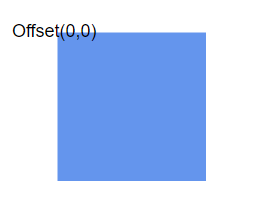(0,0.5)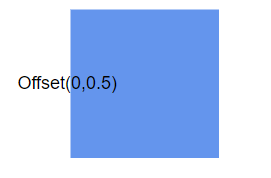(0,1)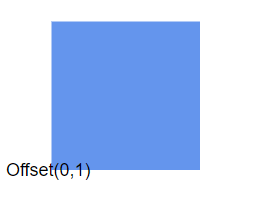(0.5,0)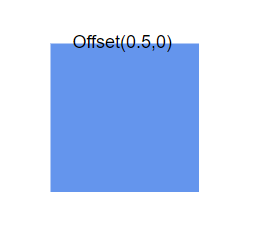(0.5,0.5)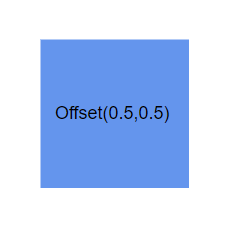(0.5,1)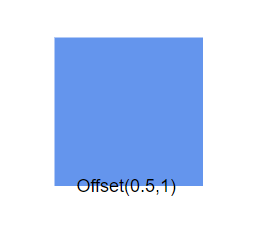(1,0)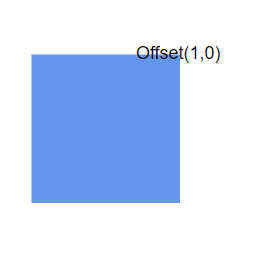(1,0.5)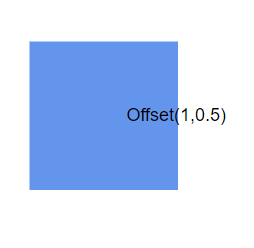(1,1)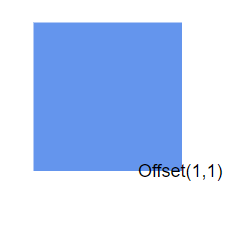• Type of the offset property for node’s shape annotation is DiagramPoint.
• Type of the offset property for connector’s path annotation is double.

## How to change the alignment of an annotation

The HorizontalAlignment property of annotation is used to set how the annotation is horizontally aligned at the annotation position determined from the fraction values. The VerticalAlignment property is used to set how the annotation is vertically aligned at the annotation position.

The following table shows all the possible alignments visually with ‘offset (0, 0)’.

Horizontal Alignment Vertical Alignment Output with Offset(0,0)
Left Top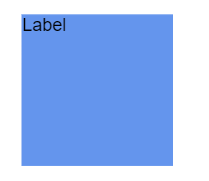Center Top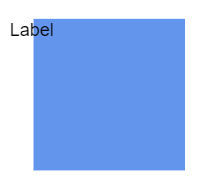Right Top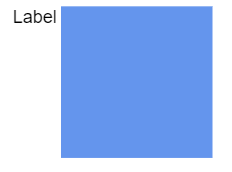Left Center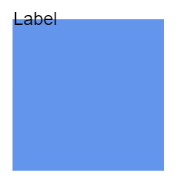Center Center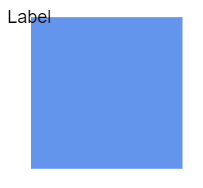Right Center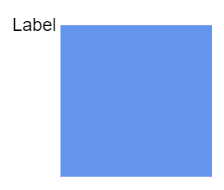Left Bottom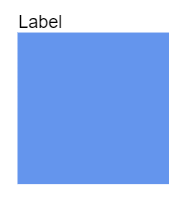Center Bottom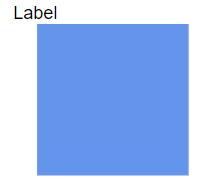Right Bottom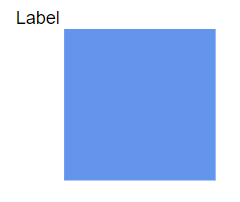The following code explains how to align annotations.

``````@using Syncfusion.Blazor.Diagram

<SfDiagramComponent Height="600px" Nodes="@nodes" />

@code
{
// Defines diagram's node collection.
DiagramObjectCollection<Node> nodes;

protected override void OnInitialized()
{
nodes = new DiagramObjectCollection<Node>();
Node node = new Node()
{
ID = "node1",
Width = 100,
Height = 100,
OffsetX = 250,
OffsetY = 250,
Annotations = new DiagramObjectCollection<ShapeAnnotation>()
{
new ShapeAnnotation
{
Content = "Annotation",
// Sets the HorizontalAlignment and VerticalAlignment for the content.
HorizontalAlignment = HorizontalAlignment.Center,
VerticalAlignment = VerticalAlignment.Center
}
},
Style = new ShapeStyle() { Fill = "#6495ED", StrokeColor = "white" },
};
}
}``````
• The value of the `HorizontalAlignment` is Center by default.
• The value of the `VerticalAlignment` is Center by default.
• Alignment positioned based on the offset value.

## How to change the margin of an annotation

Margin is an absolute value used to add some blank space to any one of its four sides. The annotations can be displaced with the margin property. The following code example explains how to align an annotation based on its Offset, HorizontalAlignment, VerticalAlignment, and Margin values.

``````@using Syncfusion.Blazor.Diagram

<SfDiagramComponent Height="600px" Nodes="@nodes" />

@code
{
// Defines diagram's node collection.
DiagramObjectCollection<Node> nodes;

protected override void OnInitialized()
{
nodes = new DiagramObjectCollection<Node>();
Node node = new Node()
{
ID = "node1",
Width = 100,
Height = 100,
OffsetX = 100,
OffsetY = 100,
Annotations = new DiagramObjectCollection<ShapeAnnotation>()
{
new ShapeAnnotation
{
// Sets the margin for the content.
Margin = new Margin() { Top = 10},
HorizontalAlignment = HorizontalAlignment.Center,
VerticalAlignment = VerticalAlignment.Top,
Offset = new DiagramPoint(){ X = 0.5 ,Y = 1}
}
},
Style = new ShapeStyle() { Fill = "#6495ED", StrokeColor = "white" },
};
}
}``````

## How to align the text

The TextAlign property of annotation allows you to set how the text should be aligned (Left, Right, Center, or Justify) inside the text block. The following code explains how to set TextAlign for an annotation.

``````@using Syncfusion.Blazor.Diagram

<SfDiagramComponent Height="600px" Nodes="@nodes" />

@code
{
// Defines diagram's node collection.
DiagramObjectCollection<Node> nodes;

protected override void OnInitialized()
{
nodes = new DiagramObjectCollection<Node>();
Node node = new Node()
{
ID = "node1",
Width = 100,
Height = 100,
OffsetX = 100,
OffsetY = 100,
Annotations = new DiagramObjectCollection<ShapeAnnotation>()
{
new ShapeAnnotation
{
Content = "Text align is set as Left",
Style = new TextStyle()
{
// Sets the textAlign as left for the content.
TextAlign = TextAlign.Left
}
}
},
Style = new ShapeStyle() { Fill = "#6495ED", StrokeColor = "white" },
};